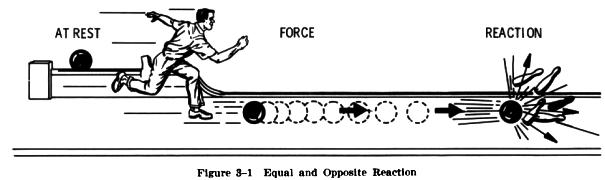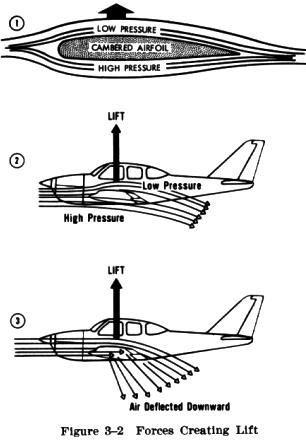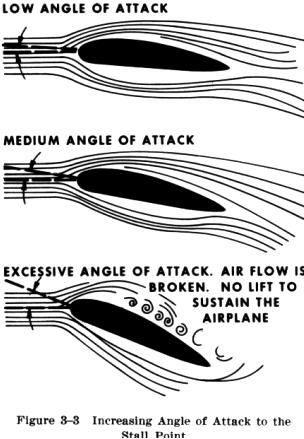Theories Theories of Flight

A balloon rises in the air because it is filled with a gas which is lighter than the air around it. But an airplane, being heavier than the air surrounding it, cannot float as does a balloon; consequently, it must get its lift in a different manner. In effect, it must do something to the air surrounding it to make the air support its greater weight. To act on the air, the airplane must be placed in motion.

The majority of general aviation type airplanes employ a reciprocating engine to turn a propeller that "bites" into the air forcing the air backward while pulling the airplane forward. This leads our discussion to several basic laws of physics that help in explaining how an airplane flies.

The English philosopher and mathematician Sir Isaac Newton is credited with having observed in 1687 that "for every action there is an equal and opposite reaction" (Fig. 3-1). This principle applies whenever two things act upon each other, such as the air and the propeller, or the air and the wing of an airplane. In short, the statement about "action and reaction" tells us how lift and propulsion of airplanes are produced.The only way air can exert a force on a solid body, such as an airplane's wing, is through pressure. In the 1700's, Daniel Bernoulli (a Swiss mathematician) discovered that if the velocity of a fluid (air) is increased at a particular point, the pressure of the fluid (air) at that point is decreased. The airplane's wing is designed to increase the velocity of the air flowing over the top of the wing as it moves through the air. To do this, the top of the wing is curved, while the bottom is relatively flat. The air flowing over the top travels a little farther (since it is curving) than the air flowing along the flat bottom. This means the air on top must go faster. Hence, the pressure decreases, resulting in a lower pressure (as Bernoulli stated) on top of the wing and a higher pressure below. The higher pressure then pushes (lifts) the wing up toward the lower pressure area (Fig. 3-2).At the same time, the air flowing along the underside of the wing is deflected downward. As stated in Newton's theorem, "for every action there is an equal and opposite reaction." Thus, the downward deflection of air reacts by pushing (lifting) the wing upward (Fig. 3-2). This is like the planing effect of a speedboat or water skier skimming over the water. To increase the lift, the wing is tilted upward in relation to the oncoming air (relative wind) to increase the deflection of air. Relative wind during flight is not the natural wind, but is the direction of the airflow in relation to the wing as it moves through the air. The angle at which the wing meets the relative wind is called the angle of attack.    These two natural forces, pressure and deflection, produce lift. The faster the wing moves through the air and the greater the two forces become, the more lift is developed. Another of Newton's theorems can be used to explain just how much air deflection is needed to lift an airplane. This law of motion says "the force produced will be equal to the mass of air deflected multiplied by the acceleration given to it." From this we can see the way lift, speed, and angle of attack are related. The amount of lift needed can be produced by moving a large mass of air through the process of making a small change in velocity, or by moving a small mass of air through the process of making a large change in velocity. For example, at a high speed where the wing affects a large amount of air, only a
small angle of attack is needed to deflect the air a small amount. Conversely, at low speeds during which the wing affects a lesser amount of air, a larger angle of attack is needed to deflect the air a large amount. Thus, the angle of attack at various speeds must be such that the deflection of air is adequate for the amount of lift needed.

 If the airplane's speed is too slow, the angle of attack required will be so large that the air can no longer follow the upper curvature of the wing. This results in a swirling, turbulent flow of air over the wing and "spoils" the lift. Consequently, the wing stalls. On most airplanes this critical angle of attack is about 15 to 20 degrees (Fig. 3-3). Stalls will be thoroughly explained in the chapter on Proficiency Flight Maneuvers.    As noted earlier, when the propeller rotates, it "bites" into the air, thereby providing the force to pull (or push) the airplane forward. This forward motion causes the airplane to act on the air to produce lift. The propeller blades, just like a wing, are curved on one side and straight on the other side. Hence, as the propeller is rotated by the engine, forces similar to those of the wing create "lift" in a forward direction. This is called thrust. Up to this point the discussion has related only to the "lifting" force. Before an understanding of how an airplane flies is complete, other forces must be discussed.